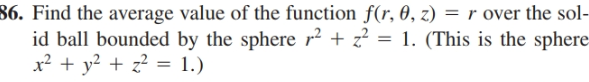# 86. Find the average value of the function f(r, 0, z) = r over the sol- id ball bounded by the sphere r + z? = 1. (This is the sphere x² + y? + z? = 1.)

Questionhelp_outlineImage Transcriptionclose86. Find the average value of the function f(r, 0, z) = r over the sol- id ball bounded by the sphere r + z? = 1. (This is the sphere x² + y? + z? = 1.) fullscreen

### Want to see this answer and more?

Experts are waiting 24/7 to provide step-by-step solutions in as fast as 30 minutes!*

*Response times may vary by subject and question complexity. Median response time is 34 minutes for paid subscribers and may be longer for promotional offers.
Tagged in
MathCalculus

### Applications of Integration